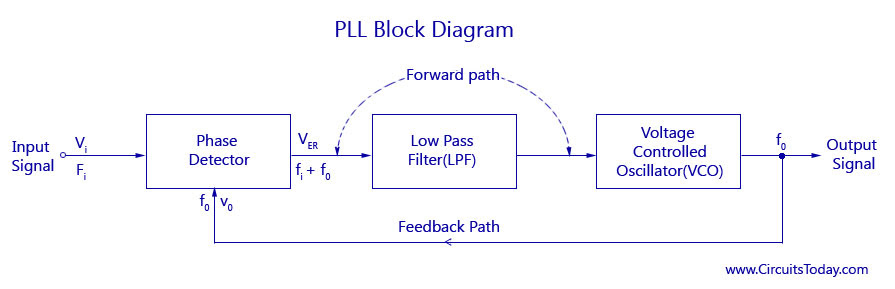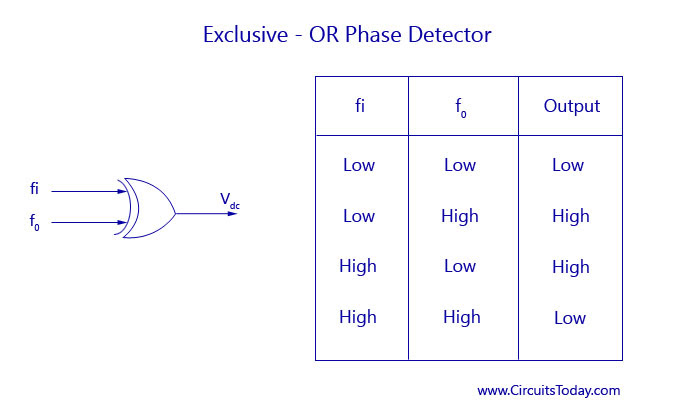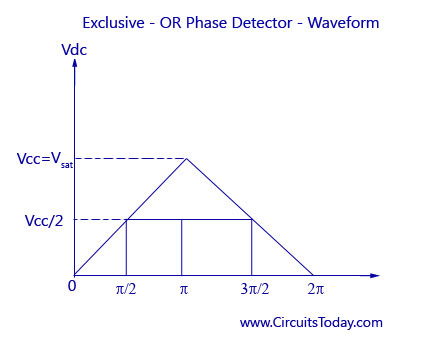Audio

[Audio][slideshow]

Phase Locked Loops (PLL)

Introduction to PLL

The concept of Phase Locked Loops (PLL) first emerged in the early 1930’s.But the technology was not developed as it now, the cost factor for developing this technology was very high. Since the advancement in the field of integrated circuits, PLL has become one of the main building blocks in the electronics technology. In present, the PLL is available as a single IC in the SE/NE560 series (560, 561, 562, 564, 565 and 567) to further reduce the buying cost ,the discrete IC’s are used to construct a PLL.

PLL Applications

• Frequency Modulation (FM) stereo decoders, FM Demodulation networks for FM operation.
• Frequency synthesis that provides multiple of a reference signal frequency.
• Used in motorspeed controls, tracking filters.
• Used in frequency shift keying (FSK) decodes for demodulation carrier frequencies.

PLL Block Diagram

The block diagram of a basic PLL is shown in the figure below. It is basically a flip flop consisting of a phase detector, a low pass filter (LPF),and a Voltage Controlled Oscillator (VCO).Block Diagram - Phase Locked Loops

The input signal Vi with an input frequency fi is passed through a phase detector. A phase detector basically a comparator which compares the input frequency fiwith the feedback frequency fo .The phase detector provides an output error voltage Ver (=fi+fo),which is a DC voltage. This DC voltage is then passed on to an LPF. The LPF removes the high frequency noise and produces a steady DC level, Vf (=Fi-Fo). Vf also represents the dynamic characteristics of the PLL.

The DC level is then passed on to a VCO. The output frequency of the VCO (fo) is directly proportional to the input signal. Both the input frequency and output frequency are compared and adjusted through feedback loops until the output frequency equals the input frequency. Thus the PLL works in these stages – free-running, capture and phase lock.

As the name suggests, the free running stage refer to the stage when there is no input voltage applied. As soon as the input frequency is applied the VCO starts to change and begin producing an output frequency for comparison this stage is called the capture stage. The frequency comparison stops as soon as the output frequency is adjusted to become equal to the input frequency. This stage is called the phase locked state.

Now let us study in detail about the various parts of a PLL – The phase detector, Low Pass Filter and Voltage Controlled Oscillator.

1. Phase Detector

This comparator circuit compares the input frequency and the VCO output frequency and produces a dc voltage that is proportional to the phase difference between the two frequencies. The phase detector used in PLL may be of analog or digital type. Even though most of the monolithic PLL integrated circuits use analog phase detectors, the majority of discrete phase detectors are of the digital type. One of the most commonly used analog phase detector is the double balanced mixer circuit. Some of the common digital type phase detectors are

1.1 Exclusive OR Phase Detector

An exclusive OR phase detector is shown in the figure below.Exclusive-OR Phase Detector

It is obtained as a CMOS IC of type 4070. Both the frequencies are provided as an input to the EX OR phase detector. Obeying the EX-OR concept the output becomes HIGH only if either of the inputs fi or fo becomes HIGH. All other conditions will produce a LOW output. Let us consider a waveform where the input frequency leads the output frequency by θ degrees. That is, fi and fo has a phase difference of θ degrees. The dc output voltage of the comparator will be a function of the phase difference between its two inputs.

The figure shows the graph of DC output voltage as a function of the phase difference between fi and fo. The output DC voltage is maximum when the phase detector is 180°.This type of phase detector is used when both fi and fo are square waves.Exclusive-OR Phase Detector-Waveform

1.2 Edge Triggered Phase Detector

Edge triggered phase detector is used when fi and fo are pulse waveforms with less than 50% duty cycles. The figure of such a phase detector using an R-S Flip Flop is shown below. Two NOR Gate (CD4001) are cross-coupled to form an R-S Flip Flop. The output of the phase detector changes it’s logic state by triggering of the R-S Flip Flop. That is, the output of the phase detector changes its logic state on the positive edge of the input fi and fo. The advantage of such a detector can be understood from the graph below. It is clear that the DC output voltage is linear over 360°.

1.3 Monolithic Phase Detectors

The monolithic type phase detector uses a CMOS type 4044 IC ,Which is highly advantages as the harmonic sensitivity and duty cycle problems are neglected and the circuit will be respond only to the transition in the input signals. This is the most preferred phase detector in the critical applications as the phase error and the output error voltage are independent of variations in the amplitude and duty cycles of the input waveforms.

2. Low Pass Filter (LPF)

A Low Pass Filter (LPF) is used in Phase Locked Loops (PLL) to get rid of the high frequency components in the output of the phase detector. It also removes the high frequency noise. All these features make the LPF a critical part in PLL and helps control the dynamic characteristics of the whole circuit. The dynamic characteristics include capture and lock ranges, bandwidth, and transient response. The lock range is the tracking range where the range of frequencies of the PLL system follows the changes in the input frequency. The capture range is the range in which the Phase Locker Loops attains the Phase Lock.

When the filter bandwidth is reduced, the response time increases .But this reduces the capture range. But it also helps in reducing noise and in maintaining the locked loop through momentary losses of signal. Two types of passive filter are used for the LPF circuit in a PLL. An amplifier is used also with LPF to obtain gain. The active filter used in PLL is shown below.

3. Voltage Controlled Oscillator (VCO)

The main function of the VCO is to generate an output frequency that is directly proportional to the input voltage. The connection diagram of a SE/NE 566 VCO is shown in the figure below. The macimum frequency of the VCO is 500 KHz.

This VCO provides simultaneous square wave and triangular wave outputs as a function of the input voltage. The frequency of oscillation is determined by the resistor R and capacitor C along with the voltage Vc applied to the control terminal.

Monolithic Phase Locked Loop

PLL is now readily available as IC’s which were developed in the SE/NE 560 series. Some of the commonly used ones are the SE/NE 560,561,562,564,565 and 567.The difference between each one of them is in the different parameters like operating frequency range, power supply requirements, and frequency and bandwidth ranges. Out of all the series the SE/NE 565 is the most famous. It is available as a 14-pin DIP and also as a 10-pin metal can package. The 14-pin DIP and its characteristics are given below.

Monolithic PLL Characteristics

• Operating frequency range: 0.001 Hz to 500 kHz.
• Operating voltage range: ± 6 to ± 12 V.
• Input impedance: 10 k Q typically.
• Output sink current: 1mA typically.
• Output source current: 10 m A typically.
• Drift in VCO centre frequency with temperature: 300 ppm/ °C typically.
• Drift in VCO centre frequency with supply voltage: 1.5 %/V maximum.
• Input level required for tracking: 10 mVrms minimum to 3 V peak-to-peak maximum.
• Bandwidth adjustment range: < ± 1 to > ± 60 %.

The block diagram and connection diagrams are shown in the figure below.

The block diagram consist of a phase detector which acts as a phase comparator, an amplifier, and a low pass filter with the combination of the resistor (3.6 kilo ohm) and capacitor C2. The output of the amplifier is fed back to the VCO. The different pins representing that of the IC are also shown in the block diagram. Pins 1 and 10 are the positive and negative supply pins. The pins 2 and 3 are the input to the phase detector. The input signals are fed through these pins in differential mode. Pin 4 is the VCO output and pin 5 is the phase comparator VCO input. If both these pins are shorted the output of the VCO is supplied back to the phase comparator. The output of the phase comparator is given to the amplifier. The amplifier has two outputs that goes to the external pins as the demodulator output (pin 7) and the reference output (pin 6). An LPF circuit is formed by connecting the capacitor C2 between pin 7 and 10 with a resistor of value 3.6 kilo ohms. The value of C2 must be large enough to eliminate the variations in demodulated output and stabilize the VCO frequency.

Pins 8 and 9 are used to connect the external resistor (R1) and external capacitor (C1). The values of R1 and C1 help to adjust the free running frequency (fr) of the PLL. Though the value of C1 can be anything, the value of resistor R1 must have a value between 2 to 20 kilo ohms. All these factors can be used to determine the center frequency of the PLL.

The free running frequency of the PLL is given as fr = (1.2)/(4R1C1) Hertz

The lock range of the PLL is given as fLock = (+/-){(8fr)/V} Hertz

The capture range of PLL is given as fc = (fLock/[2* 10^3*C2])^1/2

The lock range usually increases with an increase in input voltage but falls with an increase in supply voltage.

Let us consider the free running frequency to be fr. Let fr be the frequency at which the Voltage Controlled Oscillator (VCO) is running without input signal. Let the input signal fi that is increasing from zero be applied to the phase comparator.

A graph between the error voltage and input frequency is shown below. It can be seen that when the input frequency is lesser than fi1,the error voltage Ver is reduced to zero. At this time the VCO will operate at the free running frequency, fr. When the input frequency, fi increases and reaches fi1, the error voltage jumps from zero to a negative voltage. This value will be equal to the difference between the input frequency and actual VCO output frequency (fi – fo).This resulting error voltage is then processed by filtering, amplifying, and applying the amplified voltage Vd to the control terminals of the VCO.

The instantaneous frequency of VCO decreases because fo falls for nega­tive values of Vd and increases for positive values of Vrf. At some instant of time, the decreasing frequency of the VCO equals fin1 (lower edge of the capture range), then lock results-in, and the output signal frequency of the

VCO may be equal to the input signal frequency (that is, fo = fi). The VCO frequency locks with input signal frequency up to fi2 (the upper end of the lock range). If the input signal frequency exceeds fi2 then error voltage Vg will fall to zero and the VCO will operate at the free running frequency fr, as illustrated in figure. If the input signal frequency is now slowly swept back and it attains the value of fd1 then the loop (VCO frequency) locks with the input signal frequency, causing a positive jump of the error voltage Ver. So the VCO output frequency increases from fr continuously till fo becomes equal to fi. The VCO frequency fo locks with the input signal frequency fi upto fd2 (the lower edge of the lock range) as shown in figure by dotted lines. Now if the frequency of the input signal falls below fd2, then the error voltage Ver will fall to zero and the VCO will operate at the free running frequency.

[Lights][grids]